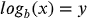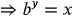1
78
views
35a
Problem

# Problem 35a

## Chapter 1: Functions and Models

###### Unlmited Homework Help for \$1 and win iPhone 13
Get a \$1 Homework+ and follow @oneclass.store on TikTok for a chance to win an iPhone 13.Textbook ExpertVerified Tutor
13 Oct 2021

#### Given information

Note that the given expression is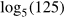.

#### Step-by-step explanation

Step 1.

The natural log of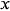raised to the power of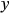is times the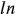of. Since the bases are the same, the two expressions are equal only if the exponents are also equal

Therefore, according to the change of base formula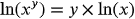Let, the expression of value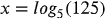Now we will apply logarithmic property to find the value of the expression,

i.e.